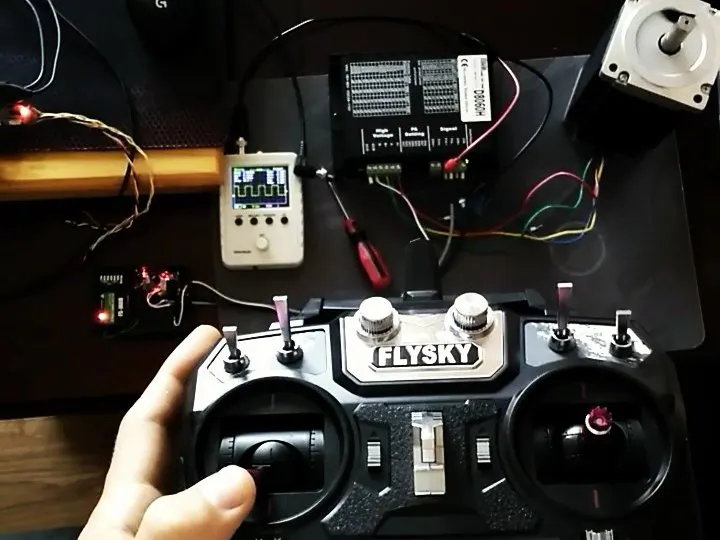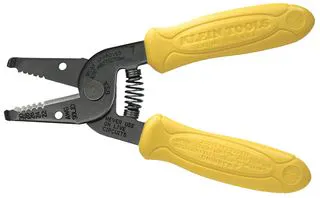# 2-Axis driver for stepper motors with the FlySky RC

The FlySky joystick is adopted to control the speed and limitations of the ramp of two stepper motors

• 5,479 views
• 1 comment
• 12 respects

## Necessary tools and machinesSoldering iron (generic)Wire Stripper & Cutter, 18-10 AWG / 0.75-4mm² Capacity Wires
 oscilloscope

## Apps and online services

Two of the stepper motors and power drivers D8060H (6A) were used for one of the camera crane control project.

In the first stage, the control is done with a simple joystick: two axes. In addition, there are two potentiometers for each of the motors to set the maximum speed and acceleration.

The remote control is battery-powered and the clock speed of the microcontroller has been lowered to 8MHz under a reduced 4.5V power supply. In addition, an automatic shutdown has been added in the absence of activity after 5 minutes. Currently, the maximum frequency of pulses to the motor (1.8deg.) controller is 200Hz.

In the second stage, the control method was practically completely rebuilt.

The generation of pulses from the CTC options of Timers 1 and 2 was created for smooth and gently moves. Frequencies from 2kHz to 16kHz were obtained. High frequencies when setting the power control on a larger number of steps per revolution allowed to obtain a gentle dynamic of the motor.

The four stage. The movement of the motors is mechanically limited. For safe stopping, the position of the motors is monitored. Added feedback from the multi-turn potentiometer for each of the two axes.

In addition, the position value is sent to the controller (telemetry) and displayed in % as temperature and the number of engine revolutions (it is not possible to change the description and unit). Arduino sends values from mapped of the analog input by receiver to the hand controller.

In the fifth stage (final stage) it is planned to add an accelerometer or gyroscope to stabilize and balance the camera.

## Code

##### Mikrokrokowy - 1 stageC/C++
arduino basic firmware for stepper motor controller
```#ifdef F_CPU
#undef F_CPU
#define F_CPU 8000000L
#endif

#define MAX_D_IMPL  9000
#define MAX_A       9000
#define LED_MRUG    500UL

#define X_GND_PIN     10
#define Y_GND_PIN     9
#define POT_VCC_PIN   3
#define LED_PIN       4
#define PRZYC_PIN     2

#define X_STEP_PIN  13
#define X_EN_PIN    12
#define X_DIR_PIN   11
#define X_OS        A6
#define X_OGR_V     A1
#define X_OGR_A     A2

#define Y_STEP_PIN  6
#define Y_EN_PIN    7
#define Y_DIR_PIN   8
#define Y_OS        A7
#define Y_OGR_V     A4
#define Y_OGR_A     A5

#define POZ_HIST_JOY_ZERO MAX_D_IMPL/40

#define SPAC    300000UL  //5 min
#include <avr/sleep.h>

uint64_t cMicr, teraz, czas_bater, czas_led;
uint64_t XprMicr, Xd_Micr, YprMicr, Yd_Micr;
int16_t Xv, Xa, Yv, Ya;
bool Xp, Yp;

void wakeUpNow()
digitalWrite(LED_PIN, HIGH);
digitalWrite(POT_VCC_PIN, HIGH);
}

void setup()
{
pinMode(X_GND_PIN, OUTPUT);
digitalWrite(X_GND_PIN, LOW);
pinMode(Y_GND_PIN, OUTPUT);
digitalWrite(Y_GND_PIN, LOW);
pinMode(POT_VCC_PIN, OUTPUT);
digitalWrite(POT_VCC_PIN, HIGH);
pinMode(LED_PIN, OUTPUT);
pinMode(PRZYC_PIN, INPUT_PULLUP);

pinMode(X_STEP_PIN, OUTPUT);
pinMode(X_EN_PIN, OUTPUT);
pinMode(X_DIR_PIN, OUTPUT);
digitalWrite(X_STEP_PIN, LOW);
digitalWrite(X_EN_PIN, LOW);
digitalWrite(X_DIR_PIN, LOW);
pinMode(X_OS, INPUT);
pinMode(X_OGR_V, INPUT);
pinMode(X_OGR_A, INPUT);

pinMode(Y_STEP_PIN, OUTPUT);
pinMode(Y_EN_PIN, OUTPUT);
pinMode(Y_DIR_PIN, OUTPUT);
digitalWrite(Y_STEP_PIN, LOW);
digitalWrite(Y_EN_PIN, LOW);
digitalWrite(Y_DIR_PIN, LOW);
pinMode(Y_OS, INPUT);
pinMode(Y_OGR_V, INPUT);
pinMode(Y_OGR_A, INPUT);
}
void sleepNow()
{
digitalWrite(LED_PIN, LOW);
digitalWrite(POT_VCC_PIN, LOW);
set_sleep_mode(SLEEP_MODE_PWR_DOWN);
sleep_enable();
attachInterrupt(0, wakeUpNow, LOW);
sleep_mode();
sleep_disable();
detachInterrupt(0);
}
void loop()
{
cMicr = micros();
teraz = millis();
// oś X
Xv = map(analogRead(X_OS), 0, 1023, -MAX_D_IMPL, MAX_D_IMPL);
if (Xv > POZ_HIST_JOY_ZERO)
{
Xv = MAX_D_IMPL - Xv + map(analogRead(X_OGR_V), 0, 1023, MAX_D_IMPL, 1);
}
else if(Xv < 0-POZ_HIST_JOY_ZERO)
{
digitalWrite(X_DIR_PIN, HIGH);
Xv += MAX_D_IMPL + map(analogRead(X_OGR_V), 0, 1023, MAX_D_IMPL, 1);
}
else
{
digitalWrite(X_EN_PIN, HIGH);
digitalWrite(X_DIR_PIN, LOW);
digitalWrite(X_STEP_PIN, LOW);
Xv=0;
Xa = map(analogRead(X_OGR_A), 0, 1023, MAX_A, 0);
digitalWrite(LED_PIN, LOW);
}
if(Xv)
{
digitalWrite(X_EN_PIN, LOW);
Xd_Micr = long(Xv + (Xa?Xa--:0));
if(cMicr - XprMicr > Xd_Micr)
{
XprMicr = cMicr;
Xp = !Xp;
digitalWrite(X_STEP_PIN, Xp);
digitalWrite(LED_PIN, Xp);
czas_bater = teraz;
}
}
// oś Y
Yv= map(analogRead(Y_OS), 0, 1023, -MAX_D_IMPL, MAX_D_IMPL);
if (Yv > POZ_HIST_JOY_ZERO)
{
Yv = MAX_D_IMPL - Yv + map(analogRead(Y_OGR_V), 0, 1023, MAX_D_IMPL, 1);
}
else if (Yv < 0-POZ_HIST_JOY_ZERO)
{
digitalWrite(Y_DIR_PIN, HIGH);
Yv += MAX_D_IMPL + map(analogRead(Y_OGR_V), 0, 1023, MAX_D_IMPL, 1);
}
else
{
digitalWrite(Y_EN_PIN, HIGH);
digitalWrite(Y_DIR_PIN, LOW);
digitalWrite(Y_STEP_PIN, LOW);
Yv=0;
Ya = map(analogRead(Y_OGR_A), 0, 1023, 0, MAX_A);
}
if(Yv)
{
digitalWrite(Y_EN_PIN, LOW);
Yd_Micr = long(Yv + (Ya?Ya--:0));
if(cMicr - YprMicr > Yd_Micr)
{
YprMicr = cMicr;
Yp = !Yp;
digitalWrite(Y_STEP_PIN, Yp);
digitalWrite(LED_PIN, Yp);
czas_bater = teraz;
}
}
if((teraz - czas_led > LED_MRUG) && !Xv && !Yv)
{
czas_led = teraz;
digitalWrite(LED_PIN, HIGH);
delay(55);
}
if(teraz - czas_bater > SPAC) sleepNow();
}
```

June 5, 2019

#### Members who respect this project

See similar projects
you might like

#### Turn your RC Car to Bluetooth RC car

Project tutorial by Prajjwal Nag

• 29,027 views
• 33 respects

#### Arduino Nano: Control 2 Stepper Motors With Joystick

Project tutorial by Boian Mitov

• 28,490 views
• 51 respects

• 26,857 views
• 52 respects

#### Arduino Motor Shield PCB V1 | 4 Motors at Once

Project tutorial by Jithin Sanal

• 5,353 views
• 15 respects

#### DC Motors Controller Board

Project showcase by Shahariar

• 4,605 views